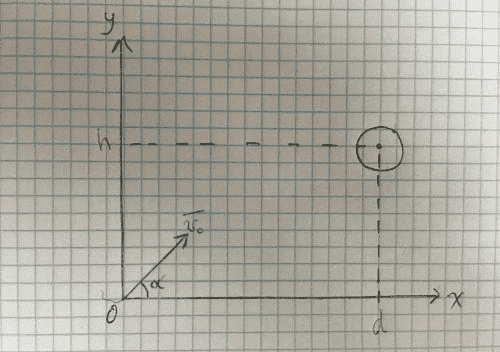# Projectile motion - determining initial velocity and angle

• marksyncm

## Homework StatementAn archer launches an arrow from coordinates ##(0, 0)## at an angle ##\alpha## and with an initial velocity ##v_0##. There's a target located ahead of the archer and the center of that target is at coordinates ##(d, h)##. At what ##v_0## and at what angle ##\alpha## does the archer need to launch his arrow to hit the center of the target perpendicular to its surface?

## Homework Equations

Kinematic equations

## The Attempt at a Solution

We have that:

##v_x = v_0\cos(\alpha)##
##v_y = v_0\sin(\alpha) - gt##

For the arrow to hit the center of the target perpendicular to its surface, it must have a vertical velocity of zero when it reaches the target. So the following conditions must be met:

1) By the time the arrow travels a distance ##d## in the ##x## direction, its vertical velocity component should be equal to zero.
2) By that same time above, the arrow should be located at height = ##h##.

So we have:

##d = v_0\cos(\alpha)t_i \rightarrow t_i = \frac{d}{v_0\cos(\alpha)}## ........ (1)
##0 = v_0\sin(\alpha) - gt_i \rightarrow t_i = \frac{v_0\sin(\alpha)}{g}## ...... (2)
##\frac{d}{v_0\cos(\alpha)} = \frac{v_0\sin(\alpha)}{g} \rightarrow v_0^2\sin(\alpha)\cos(\alpha) = dg## .... (3)

Where ##t_i## is the time of impact.

I'm at a loss as to how to proceed from here. I'm assuming I need to use the displacement equation in the vertical direction: ##h = v_0\sin(\alpha)t - \frac{g}{2}t^2##, but I am not sure what to put into this equation. Do I put in the ##t_i## from equation (1) or from equation (2)? Can I use both values of ##t_i## simultaneously (insert one under ##t## and another under ##t^2##) since they are supposed to be the same thing? I tried doing this and I get an algebraic expression that I'm unable to process. For example, here's what happens when I use ##t_i## from equation (1):

$$h=\frac{v_0\sin(\alpha)d}{v_0\cos(\alpha)} - \frac{gd^2}{2v_0^2\cos^2(\alpha)} \rightarrow h = \frac{sin(\alpha)}{\cos(\alpha)} - \frac{gd^2}{2v_0^2cos^2(\alpha)}$$

But this equation doesn't account for the fact that at time ##t_i##, the vertical velocity needs to be zero. How do I proceed?

#### Attachments

Your image suggests that the target is a sphere, but your solution and the formulation suggests that it is a vertical surface. I am going to assume the latter.

The value of ##t_i## must be the same in both cases. If not, your conditions would not be satisfied. However, I would suggest a different approach than using ##v_0## and ##\alpha## from the beginning. Instead, I would use the initial ##x## and ##y## components of the velocity (call them ##v_{0x}## and ##v_{0y}## for example). Either way, you should have a sufficient number of conditions to solve for all of your variables. In principle, you have three conditions and three unknowns:
1. The vertical velocity needs to be zero at ##t_i##.
2. The vertical distance needs to be ##h## at ##t_i##.
3. The horizontal distance needs to be ##d## at ##t_i##.
This is a solvable system of equations.

•marksyncm
Your image suggests that the target is a sphere, but your solution and the formulation suggests that it is a vertical surface. I am going to assume the latter.

Sorry for the confusion. Your assumption is correct.

Thanks, I'll take another stab at the problem using your approach and will see how it goes.

Here's what I did:

Time when vertical velocity = 0:

$$0 = v_{0y} - gt_i \rightarrow t_i = \frac{v_{0y}}{g}$$

Vertical distance is ##h## at time = ##t_i##:

$$h = v_{0y}t - \frac{g}{2}t^2 \rightarrow H=v_{0y}\frac{v_{0y}}{g} - \frac{g}{2}\frac{v_{0y}^2}{g^2} = \frac{v_{0y}^2}{g}-\frac{v_{0y}^2}{2g} = \frac{v_{0y}^2}{2g}=h$$

Horizontal distance is ##d## at time = ##t_i##:

$$d=v_{0x}t \rightarrow d=v_{0x}\frac{v_{0y}}{g} \rightarrow d=\frac{v_{0x}v_{0y}}{g}$$

So we have two equations:

##h = \frac{v_{0}^2 sin^2(\alpha)}{2g}## ...... (1)
##d=\frac{v_{0}^2 \sin \alpha cos \alpha}{g}## ...... (2)

From equation (2), we get that ##v_{0}^2 = \frac{dg}{\sin \alpha \cos \alpha}##. Substituting this into equation (2):

$$h = \frac{dg \sin^2(\alpha)}{2g\sin(\alpha)\cos(\alpha)} = \frac{d\sin(\alpha)}{2\cos(\alpha)} = \frac{d}{2} \tan(\alpha) = h \rightarrow \frac{2h}{d} = \tan(\alpha) \iff \alpha = tan^{-1}(\frac{2h}{d})$$

Is this correct?

However, I'm not sure how to get the value for ##v_0## from equations (1) and (2). It seems that whatever substitution I make ends up with a ##v_0^2## in the numerator canceling out with another ##v_0## in the denominator. Would appreciate a tip.

Well, ##\alpha## is now known so you can just substitute it into either (1) or (2) and solve for ##v_0##.

Edit: ... or you could just solve for ##v_{0x}## and ##v_{0y}## and apply Pythagoras' theorem.

•marksyncm
Well, ##\alpha## is now known so you can just substitute it into either (1) or (2) and solve for ##v_0##.

Edit: ... or you could just solve for ##v_{0x}## and ##v_{0y}## and apply Pythagoras' theorem.

Thank you!

Is it "unusual" that I cannot find the value of ##v_0## from just equations (1) and (2) (without substituting in ##\alpha##)? Or am I just not seeing a way to do it?

No, it is not unusual in any way because ##v_0## has contributions from both the vertical and the horizontal velocity components.

•marksyncm
Thank you!

Is it "unusual" that I cannot find the value of ##v_0## from just equations (1) and (2) (without substituting in ##\alpha##)? Or am I just not seeing a way to do it?
You can use the trigonometric identity ##\sin^2(α)=\frac{\tan^2(α)}{1+\tan^2(α)}## in equation (1).
Or you can apply the double-angle formulas sin2(α)=(1-cos(2α))/2, sin(α)cos(α)=sin(2α)/2,
isolate sin(2α) and cos(2α), square and add the squares, resulting 1. You get an equation for v02.

Last edited:
I think I would have begun with conservation of energy to get the vertical velocity component:

##\frac{1}{2}v_y^2 = gh## so that ##v_y = \sqrt{2 g h}##

Then, since the time to rise to the maximum height is the same as for falling from that height,

##\frac{1}{2} g t^2 = h## so that ##t = \sqrt{2 \frac{h}{g}}##

##v_x## then follows from the time and horizontal distance. The angle follows that from ##\arctan(\frac{v_y}{v_x})##.